• 矩阵乘法* 比如说 p = a * inv(a) # a乘以逆矩阵 4.显示数据保留小数位数 format long format short 5.串联(也就是拼接两个矩阵) 横向拼接: A= [a, a] 纵向拼接: B = [a; a] 6.复数使用符号i 或者j 7.不会立刻输出...

数组创建

1.要创建每行包含四个元素的数组，请使用逗号 (,) 或空格分隔各元素。

这种数组为行向量

要创建包含多行的矩阵，请使用分号分隔各行。

a = [1 2 3; 4 5 6; 7 8 10]

2.创建矩阵的另一种方法是使用 oneszeros 或 rand 等函数。例如，创建一个由零组成的 5×1 列向量。

z = zeros(5,1)

处理数组

1.使用数学符号处理矩阵中的每个数字

a + 10

每个数字+10

2.转置 a'

3.矩阵乘法*

比如说 p = a * inv(a)

# a乘以逆矩阵

4.显示数据保留小数位数

format long

format short

5.串联(也就是拼接两个矩阵)

横向拼接: A= [a, a]

纵向拼接: B = [a; a]

6.复数使用符号i 或者j

7.不会立刻输出结果就用;结尾

8.保存结果到变量中 xx = xxxxx

不保存的话 直接输入代码 xxx

9.输入太长的话不换行, 但是想要换行显示 空格+...+,

展开全文matlab
• MATLAB基本运算单元为矩阵，所以我们需要了解对矩阵的一些简单的语法操作。 1、如何生成一个矩阵（来自百度） （1）元素输入法 （2）设定步长生成 形式为： A=a:inc:b 可以看出a为初值，inc为步长...

MATLAB基本运算单元为矩阵，所以我们需要了解对矩阵的一些简单的语法操作。

1、如何生成一个矩阵（来自百度）

（1）元素输入法（2）设定步长生成

形式为： A=a:inc:b可以看出a为初值，inc为步长，b为终值（界限）    相当于生成了一个等差数列

注意当省略步长的时候，默认步长为1（3）均匀采样生成

格式为   A=linspace(a,b,n)可以看出，a为初值，b为终值，n为分成的份数，也是生成了一个等差数列

（4）特殊矩阵的生成方法

•              A=[] %空矩阵
•              A=zeros(2,3) %生成元素全为零的2x3矩阵
•              A=ones(2,3)  %生成元素全为1的2x3矩阵
•              A=rand(2,4)  %生成随机的2x5矩阵
•     矩阵的提取（ps:矩阵提取还可以通过其他方式提取，通过逻辑矩阵，暂时不介绍）：

A=[1:5;6:10;3:7]

B=A(2:3,2:4)  %提取矩阵A的第2,3行与第2,3,4列交叉处的元素。

•               A=magic（3）  %创建魔方矩阵。
•               A=eye(3,4)      %使用eye(m,n)可得到一个允许的最大单位矩阵，其余处补0。

此处特别讲解一下矩阵的提取

涉及到冒号：的使用，之前其实已经讲过先举一个简单的例子现在想提取位于第2-3行  第2-4列的元素当然也可以隔行隔列提取如果想要提取所有的行，第1 3 5列的元素这里还有一个技巧：

end的使用用于提取矩阵归纳：B=A(i,j)

i  j可以是数   也可以是向量

当i 为向量时，比如i=[1 2 4];  即提取A的第1  2  4行    j同理

ps:   还有一种常用的提取矩阵元素的方法

当A为二维矩阵有如下方法：想必大家对于第二种方法有疑问，这里就涉及到MATLAB中矩阵存储形式了，不管怎么样，计算机是串行存取数据的，

那么矩阵又是如何存储的，肯定要先按成一维向量存取，最后知道分界线即可！！！（个人猜测）然后介绍一些矩阵的简单操作基本上这些，剩余对矩阵操作的命令都可以百度的，哈哈

转载于:https://www.cnblogs.com/try-nocatch/p/7253512.html

展开全文• matlab矩阵操作

2012-05-21 09:35:52
matlab矩阵操作语法，对初学者有帮助！比较实用matlab
• Matlab矩阵大全

千次阅读 2020-10-07 00:19:25
目录 1.矩阵下标引用 2.矩阵合并 3、矩阵运算（加、减、乘、除、点乘、点除等...4.Matlab平台提供了大量的常用的运算函数 5.生成对角矩阵的基本用法 6、生成三对角线上元素相同的矩阵 7.m行n列的元素都为0的矩阵

最近的项目需要一些矩阵的语法，现汇总如下（后续有时间的话，会继续补充）：

目录

1.矩阵下标引用

2.矩阵合并

3、矩阵运算（加、减、乘、除、点乘、点除等）

4.Matlab平台提供了大量的常用的运算函数

5.生成对角矩阵的基本用法

6、生成三对角线上元素相同的矩阵

7.m行n列的元素都为0的矩阵

1.矩阵下标引用

表达式(Matlab程序)  函数功能
A(1)将二维矩阵A重组为一维数组，返回数组中第一个元素
A(: , j)返回二维矩阵A中第 j 列 列向量
A( i , :)返回二维矩阵A中第 i 行 行向量
A(: , j : k)返回二维矩阵A中第 j 列第 k列 列向量组成的子矩阵
A( i : k , :)返回二维矩阵A中第 i 行第 k行 行向量组成的子矩阵
A( i : k , j : m)

返回二维矩阵A中第 i 行第 k 行 行向量

第 j 列第 m 列 列向量的交集组成的子矩阵

A(:)将二维矩阵A中得每列合并成一个列向量
A( j : k)返回一个行向量，其元素为A(:)中的第 j 个元素到第 k 个元素
A([ j1 j2…])返回一个行向量，其元素为A(:)中的第 j1，j2…个元素
A(: , [ j1 j2 …])返回矩阵A的第 j1 列、第 j2 列等的列向量
A([ i1 i2 …] : ,)返回矩阵A的第 i1 行、第 i2 行等的行向量
A([ i1 i2 …] , [ j1 j2 …])返回矩阵A的第 j1列、第 j2 列等和矩阵A的第 i1 行、第 i2 行等的元素

下面将常用的几个举例说明：

例如：

A=[1   2 3   4  5;
12 12 14 56 657;
23 46 34 67 56 ];

（1）将二维矩阵A转化成一维矩阵（列向量）：Matlab 默认将其转化成列向量，需要行向量转置即可

Matlab程序:

A(:)  %将二维矩阵其转化成列向量

（2）读取矩阵取前N行或N列的方法

Matlab程序：

A(1:2,:)  %读取矩阵A前2行
A(:,1:3)  %读取矩阵A前3列

（3）求矩阵中每行或每列的最大值和最小值

① 找矩阵A每列的最大值

[max_A,index]=max(A,[],1);

其中，max_A是最大的数值，index是最大的数值所处的位置

② 找矩阵A每行的最大值

[max_A,index]=max(A,[],2);
其中，max_A是最大的数值，index是最大的数值所处的位置

同理可求出每行，每列的最小值。

③ 找矩阵A每列的最小值

[min_A,index]=min(A,[],1);
其中，min_A是最小的数值，index是最小的数值所处的位置

④ 找矩阵A每行的最小值

[min_A,index]=min(A,[],2);
其中，min_A是最小的数值，index是最小的数值所处的位置

2.矩阵合并

已知矩阵：

A=[1   2  3  4  5;
12 12 14 56 657;
23 46 34 67 56];
B=[1 1 1 1 1;
2 2 2 2 2;
3 3 3 3 3];

（1）矩阵A,B左右合并

horzcat(A,B); %矩阵A,B左右合并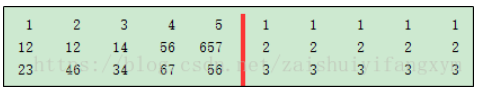（2）矩阵A,B上下合并

vertcat(A,B); %矩阵A,B上下合并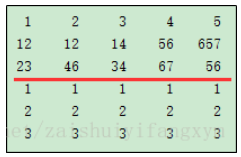3、矩阵运算（加、减、乘、除、点乘、点除等）

（1）A+B; 表示矩阵A和矩阵B相加（各个元素对应相加）；
（2）A-B; 表示矩阵A和矩阵B相减（各个元素对应相减）；
（3）A*B; 表示矩阵A和矩阵B相乘；
（4）A.*B; 表示矩阵A和矩阵B对应元素相乘（点乘）；
（5）A/B; 表示矩阵A与矩阵B相除法；
（6）A./B; 表示矩阵A和矩阵B对应元素相除（点除）；
（7）A^B; 表示矩阵A的B次幂；
（8）A.^B; 表示矩阵A的每个元素的B次幂。

4.Matlab平台提供了大量的常用的运算函数

 函数 运算法则 exp(x) 求以e为底数的x次幂 log(x) 求以e为底数对x值取对数 Log10(x) 求以10为底数x值取对数 sqrt(x) 求x的平方根 sin(x) 正弦函数 cos(x) 余弦函数 tan(x) 正切函数 asin(x) 反正弦函数 acos(x) 反余弦函数 atan(x) 反正切函数 mode(a,b) a与b相除取余数 min(a,b) 返回a, b中较小的数值 max(a,b) 返回a, b中较大的数值 mean(x) 求x的列平均数（列平均） median(x) 求x的列中位数（列中位数） sum(x) x中各个列之间的元素求和 rank(x) X矩阵的秩

5.生成对角矩阵的基本用法

（1）diag(a)
使用diag(a)命令生成对角矩阵，a为某个向量，如下所示：

A=diag([1 2 3])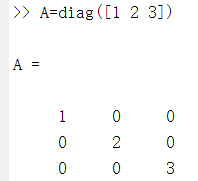（2）diag(a,i)
使用diag(a,i)命令生成，a为某个向量，i为a向量相对主对角线偏移的列数向上为正，向下为负）。当i=0时，可以直接写成diag(a)。具体情况如下：

A=diag([1 2 3],1)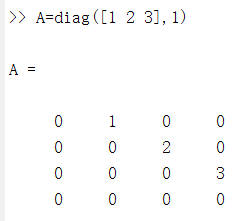A=diag([1 2 3],-1)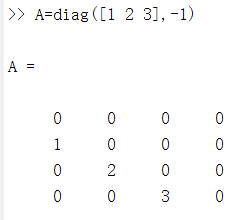6、生成三对角线上元素相同的矩阵

（1）生成全为1的向量如下：

• a(1:3,1)=1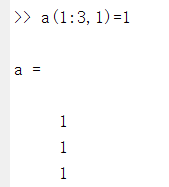• a=repmat(,3,1)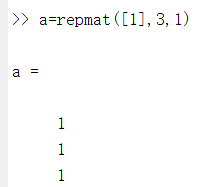注意：a=repmat(A,m,n)是用A矩阵铺成m*n块且每一块都是A的矩阵，如下：

• >> A=[1,2;3,4];
>> a=repmat(A,2,3)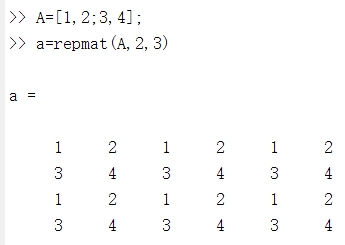• ones(3,1)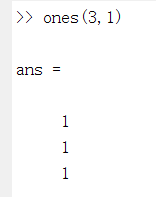（2）生成对角线上元素相同的矩阵

A=diag(repmat(,1,5))+diag(repmat(,1,4),1)+diag(repmat(,1,4),-1)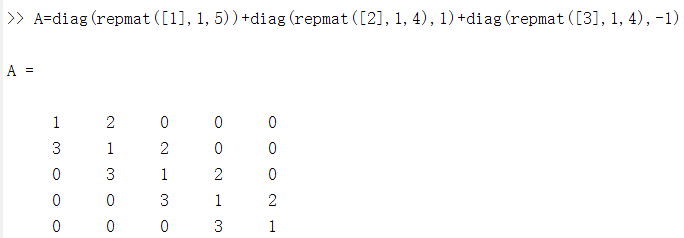7.m行n列的元素都为0的矩阵

A=zeros(3,2)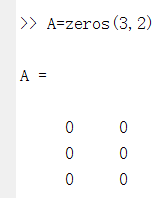后续继续补充。。。。

展开全文matlab
• end使用类似于以下内容的常规MATLAB语法：mat = C * S(1:length(S))这给出了"内部矩阵尺寸必须一致误差"，因为它正在尝试执行正常的矩阵运算。 这不是标准的线性代数运算，所以我不确定如何在...

有没有一种方法可以在MATLAB中组合2个向量，例如：

mat = zeros(length(C),length(S));

for j=1:length(C)

mat(j,:)=C(j)*S;

end

使用类似于以下内容的常规MATLAB语法：

mat = C * S(1:length(S))

这给出了"内部矩阵尺寸必须一致误差"，因为它正在尝试执行正常的矩阵运算。 这不是标准的线性代数运算，所以我不确定如何在MATLAB中正确表达它，但是似乎不需要循环就可以实现，而这在MATLAB中太慢了。

我想你有错字。" mat(1，:) = ..."行将继续覆盖第一行，而对其余行不执行任何操作。 您是说说" mat(j，:) = ..."吗？

是的，您说对了，应该是mat(j，:)。 对于那个很抱歉。

没问题。 我已修复它，以避免造成任何进一步的混乱。 =)

根据您的描述，这听起来像是简单的矩阵运算。您只需要确保C和S的尺寸正确即可。C应该是列向量(length(C)-1)，而S应该是行向量(1-length(S))。假设它们是正确的尺寸，请执行以下操作：

mat = C*S;

如果您不确定它们的尺寸，可以使用以下方法：

mat = (C(:))*(S(:)');

编辑：实际上，我对括号有点疯狂。其中一些是不必要的，因为没有操作顺序方面的问题。这是一个更干净的版本：

mat = C(:)*S(:)';

说明：

MATLAB中的矩阵乘法运算符将根据要应用的向量的尺寸来产生内积(导致标量值)或外积(导致矩阵)。

上面的最后一个方程产生一个外积，这是因为使用了冒号运算符来重塑矢量自变量的维数。语法C(:)将C的内容重塑为单个列向量。语法S(:)'将S的内容重塑为列向量，然后将其转置为行向量。乘以后，将得到大小(长(C)乘长(S))的矩阵。

注意：冒号运算符的这种用法适用于任何维数的向量和矩阵，从而使您可以将它们的内容重塑为单个列向量(如另一个SO问题所示，这使某些操作更加容易)。

为什么底部表达式与顶部表达式产生不同的结果？似乎C = C(:)。我不明白这里有些微妙的事情。

@Pete：我会在答案中添加一个解释，以使其更清楚。

如果您的说法正确，那么我会读懂您的意思，然后C * S应该起作用，并且可以观察到它确实起作用。因此，真正的问题只是列向量与行向量相乘的顺序。甜。谢谢。

乐意效劳。 C和S必须都是行向量开头，在这种情况下C * S和C(：)* S做同样的事情。实际上，只有在您不知道C和S是行向量还是列向量，但仍然想与它们执行外积的情况下，才真正需要更通用的C(：)* S(:)。

我一直认为括号是"索引运算符"，而C(：，x)是C(1：length(C)，x)的简写。文档中确实强调了操作arent的精妙之处。有点可笑，他们比语言本身更好地记录了图书馆。

括号是索引运算符的一种类型，括号之间的参数可以包含表达式和其他运算符。在括号内使用冒号运算符时，可以戴很多帽子。它可以用来制作像" C(1：length(C)，x)"这样的向量，它可以索引像" C(：，x)"这样的整个维度，或者可以按列方式访问所有元素如" C(:)"。确实，Ive发现MATLAB的文档非常好，而且通常比其他语言更清晰。

尝试在MATLAB中执行此操作：

mat = C*S'

如：

C = [1; 2; 3];

S = [2; 2; 9; 1];

mat = zeros(length(C),length(S));

for j=1:length(C)

mat(j,:)=C(j)*S;

end

% Equivalent code:

mat2 = C*S';

myDiff = mat - mat2

您是说以下意思吗？

mat = zeros(length(C),length(S));

for j=1:length(C)

mat(j,:)=C(j)*S;

end

如果是这样，那只是矩阵乘法：

C' * S    % if C and S are row vectors

C * S'    % if C and S are column vectors

如果您不知道C和S是行向量还是列向量，则可以使用技巧将它们转换为列向量，然后在乘以它们之前转置S：

C(:) * S(:)'

尝试这个：

C = 1：3

S = 1：5

mat1 = C'* S

mat2 = bsxfun(@times，C'，S)

(特别是当您需要的功能不是更简单的MATLAB表示法时很好)

-罗恩

我不清楚您在做什么-看来您生成的矩阵将由length(C)行组成，其中第i行是由i项的i比例缩放的向量S x4>(因为对向量进行下标给出了标量)。在这种情况下，您可以执行以下操作

mat = repmat(C,[1 length(S)]) .* repmat(S, [length(C) 1])

在其中将C跨列平铺，并将S向下平铺。

尝试使用meshgrid：

[Cm, Sm] = meshgrid(C, S);

mat = Cm .* Sm;

编辑：没关系，矩阵乘法也可以。您只需要一个列向量C和一个行向量S。然后执行C *S。

展开全文• NumPy和Matlab不一样，对于多维数组的运算，缺省情况下并不使用矩阵运算，可以调用相应的函数对数组进行矩阵运算。或者使用numpy库提供了的matrix类，用matrix类创建的是矩阵对象，它们的加减乘除运算缺省采用矩阵...python matlab matrix numpy 矩阵
• Matlab矩阵常用操作

万次阅读 2017-12-21 11:00:41
MATLAB中创建矩阵有以下规则： a、矩阵元素必须在”[ ]”内； b、矩阵的同行元素之间用空格（或”,”）隔开； c、矩阵的行与行之间用”;”（或回车符）隔开； d、矩阵的元素可以是数值、变量、表达式...matlab 基本操作
• matlab矩阵求逆的源码Armadillo：用于线性代数和科学计算的 C++ 库 版权所有 2008-2020 Conrad Sanderson () 版权所有 2008-2016 澳大利亚国家 ICT (NICTA) 版权所有 2017-2020 阿罗约联盟版权所有 2017-2020 Data61...
• matlab矩阵之间的运算

千次阅读 2019-09-04 22:16:08
matlab里面创建矩阵用[]即可，对于矩阵的连接运算本人也是新手，与大家分享； 最简单的方法就是使用[]进行连接； 比如矩阵A以及矩阵B进行行（hang）的连接只需要C=[A B]即可（首先保证两个矩阵的行相同）进行列的...matlab 矩阵
• matlab矩阵转置命令

千次阅读 2021-04-18 06:01:59
matlab矩阵的转置怎么表示matlab中， 矩阵A的转置表示成 A' 【附注】matlab中常用的矩阵运算矩阵加、减(＋,－)运算 规则： (1)相加、减的两矩阵必须有相同的行和列两矩阵对应元素相加减； (2)允许参与运算的两矩阵...
• 关于矩阵索引的基础知识 索引相关知识在matlab常会出现，不熟悉常会致后续程序阅读出现误解 学习一段时间后，虽然有了框架基础，但是对该方面理解非常容易遇到阻碍， 遂决定做一篇总结 基本概念 冒号表达式 ...matlab 编程语言 矩阵
• matlab矩阵求逆的源码Armadillo：用于线性代数和科学计算的 C++ 库 版权所有 2008-2018 Conrad Sanderson () 版权所有 2008-2016 澳大利亚国家 ICT (NICTA) 版权所有 2017-2018 阿罗约联盟版权所有 2017-2018 Data61...
• matlab基础语法

2021-04-18 14:31:50
title: matlab基础语法date: 2019-08-19 08:53:16一、变量变量命名：区分大小写，必须以字母开头，后可跟下划线和数字变量类型：全局变量：global X_Val,变量名大写，在函数体开头位置进行定义永久变量：persistent ...
• MATLAB中, 变量和常量的标识符最长允许 19 个字符 , 标识符中第一个字符必须是英文 字母MATLAB区分大小写 默认状态下 A 和 a 被认为是两个不同的字符 case sensitive 一数组和矩阵 ( 一数组的赋值 数组是指一组...
• 1. 数组和矩阵数组：用空格或者逗号间隔数组元素，用方括号括起来构造数组的方法：增量法和linspace函数法增量法：>> b=10:2:15b =10 12 14>> a=10:15a =1011 12 1314 15>> b=3.3:5.5b =3.30004....
• matlab矩阵代数学习笔记

万次阅读 2020-04-30 11:12:14
MATLAB中求解矩阵行列式的函数是det。 逆 MATLAB中可以通过函数inv求解矩阵的逆。 矩阵的逆在求解线性方程组时是重要的，对于一般的给定线性方程组A*X=b，其解就可以通过X=inv(A)*b求得。 需要注意的是，对于...matlab
• Matlab基础操作系列：二、文件夹读取、删除 说明：本系列文章只是记录我解决问题过程中使用的简单语法，方便日后查阅，以解决问题为主要目的，不代表实现该目的只有这一种方法，大家可以多多探索，文章仅供参考交流...matlab
• 以及将python程序转换为matlab程序，发现matlab用于计算最牛逼，python稍微差点，java那是真的恶心，特别是double类型的计算有精度丢失问题，还有引用传递，如果用java进行数学计算，特别是矩阵计算，特别要注意这两...
• MATLAB基本语法

2020-12-22 04:47:44
点乘运算,常与其他运算符点乘运算,常与其他运算符联合使用(如.\)矩阵生成 矩阵生成 向量生成或子阵提取本节将会介绍一些MATLAB的基本语法的使用。在 MATLAB 环境下进行的操作就像是使用一个超级复杂的计算器，不要被...
• matlab矩阵相关基本操作

千次阅读 2016-09-08 20:49:13
1.初始化行向量和列向量和矩阵：    行向量“a=[1 2 3]”  列向量“a=[1 2 3]’”或是“a=[1;2;3]”.  2*2矩阵“a=[1 2;3 4]”       2生成矩阵的函数       linspace(1,100,50)1到100等...
• 总结一下matlab矩阵的索引方式 a= [1 2 3 4 5 6 7 8 9 10 1 2 3 4 5 6 7 8 9 10 1 2 3 4 5 6 7 8 9 10 1 2 3 4 5 6 7 8 9 10 1 2matlab
• matlab矩阵共旁置代码CS 422 的最终项目 - 编程语言设计 这是我在 2021 年Spring参加 CS 422 时提交的最后一个项目。简而言之，它是一种简单语言（称为 SIMPLE）的扩展，它将向量和矩阵添加为内置类型。 还添加了对...
• matlab里面矩阵乘法的运算代码mtimesx 具有多维支持的快速矩阵乘法 MTIMESX是一种快速通用矩阵和标量乘法例程，具有以下功能： 直接支持多维（nD，n> 2）数组 支持移调，共轭转置和共轭预运算 支持单例扩展 利用BLAS...
• 矩阵运算matlab入门——矩阵运算1、矩阵加法2、矩阵减法3、矩阵乘法3.1、 数乘运算3.2、 矩阵相乘3.2、点乘运算4、矩阵除法4.1、左除法4.2、右除法5、矩阵其它运算汇总6、矩阵幂函数6、矩阵求逆7、矩阵范数、两个点...matlab入门 matlab基础知识 矩阵运算 matlab
• (casesensitive)一、数组和矩阵(一)数组的赋值数组是指一组实数或复数排成的长方阵列。它可以是一维的“行”或“列”，可以是二维的“矩形”，也可以是三维的甚至更高的维数。在MATLAB中的变量和常量都代表数组，...
• 我有一个八度的大矩阵,我需要将它的数据导入我的C代码.矩阵是所有数字,我想将它保存为头文件中的C数组.例如：> # octave:results =-3.3408e+01 -5.0227e+00 4.3760e+01 3.2487e+01 1.0167e+01 4.1076e+01 6.3226e...
• Matlab基本语法 matlab工作界面基本介绍： 1.新建脚本:生成*.m文件，同时打开编辑器； 2.打开：打开文件夹中的m文件； 3.绘图窗口：利用你已有的数据可以进行绘图 （后期可能会更新具体操作，尽请期待…）; 4.两个...
• MATLAB中的矩阵索引作者：SteveEddins and Loren Shure 译：王茂春利用矩阵的索引取出原矩阵的子集元素是一种有效的方式。MATLAB的多种索引类型不仅强大、灵活，而且表达清晰易读。在理解电脑MATLAB编程方面，体会......

matlab矩阵语法matlab 订阅